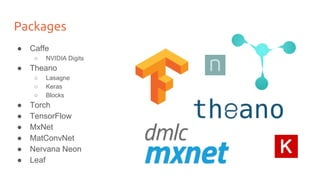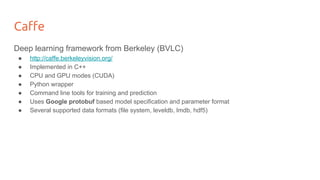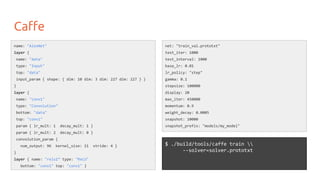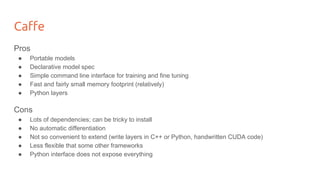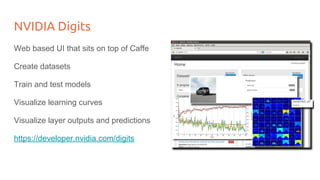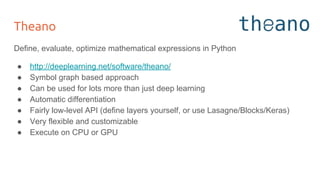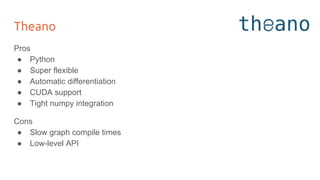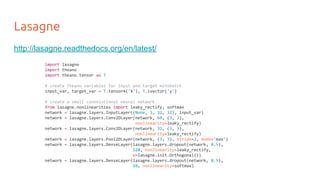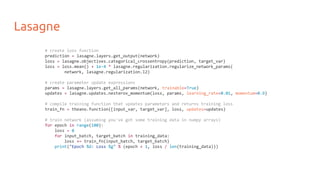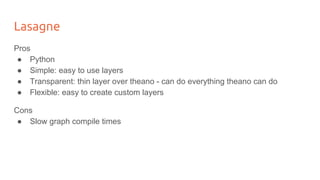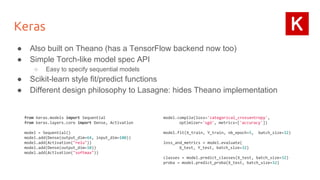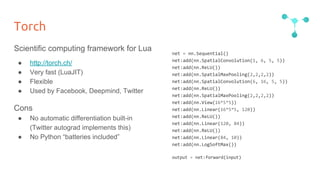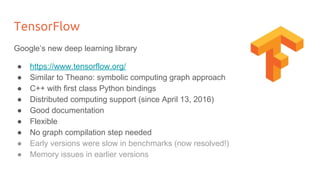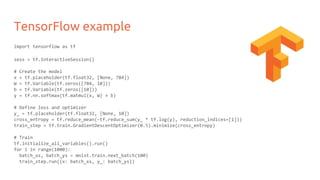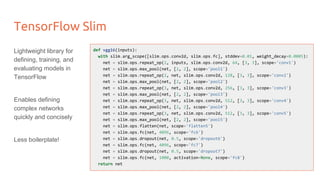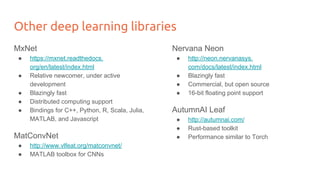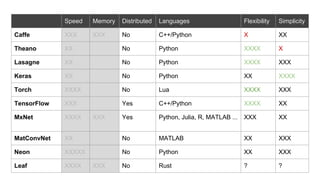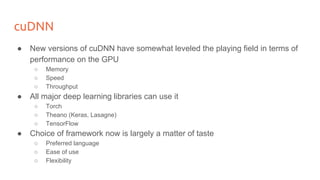1 of 19

### Deep Learning for Computer Vision: Software Frameworks (UPC 2016)

1. [course site] Software Frameworks for Deep Learning Day 1 Lecture 6 Kevin McGuinness kevin.mcguinness@dcu.ie Research Fellow Insight Centre for Data Analytics Dublin City University
2. Packages ● Caffe ○ NVIDIA Digits ● Theano ○ Lasagne ○ Keras ○ Blocks ● Torch ● TensorFlow ● MxNet ● MatConvNet ● Nervana Neon ● Leaf
3. Caffe Deep learning framework from Berkeley (BVLC) ● http://caffe.berkeleyvision.org/ ● Implemented in C++ ● CPU and GPU modes (CUDA) ● Python wrapper ● Command line tools for training and prediction ● Uses Google protobuf based model specification and parameter format ● Several supported data formats (file system, leveldb, lmdb, hdf5)
4. Caffe name: "AlexNet" layer { name: "data" type: "Input" top: "data" input_param { shape: { dim: 10 dim: 3 dim: 227 dim: 227 } } } layer { name: "conv1" type: "Convolution" bottom: "data" top: "conv1" param { lr_mult: 1 decay_mult: 1 } param { lr_mult: 2 decay_mult: 0 } convolution_param { num_output: 96 kernel_size: 11 stride: 4 } } layer { name: "relu1” type: "ReLU" bottom: "conv1" top: "conv1" } \$ ./build/tools/caffe train --solver=solver.prototxt net: "train_val.prototxt" test_iter: 1000 test_interval: 1000 base_lr: 0.01 lr_policy: "step" gamma: 0.1 stepsize: 100000 display: 20 max_iter: 450000 momentum: 0.9 weight_decay: 0.0005 snapshot: 10000 snapshot_prefix: "models/my_model"
5. Caffe Pros ● Portable models ● Declarative model spec ● Simple command line interface for training and fine tuning ● Fast and fairly small memory footprint (relatively) ● Python layers Cons ● Lots of dependencies; can be tricky to install ● No automatic differentiation ● Not so convenient to extend (write layers in C++ or Python, handwritten CUDA code) ● Less flexible that some other frameworks ● Python interface does not expose everything
6. NVIDIA Digits Web based UI that sits on top of Caffe Create datasets Train and test models Visualize learning curves Visualize layer outputs and predictions https://developer.nvidia.com/digits
7. Theano Define, evaluate, optimize mathematical expressions in Python ● http://deeplearning.net/software/theano/ ● Symbol graph based approach ● Can be used for lots more than just deep learning ● Automatic differentiation ● Fairly low-level API (define layers yourself, or use Lasagne/Blocks/Keras) ● Very flexible and customizable ● Execute on CPU or GPU
8. Theano Pros ● Python ● Super flexible ● Automatic differentiation ● CUDA support ● Tight numpy integration Cons ● Slow graph compile times ● Low-level API
9. Lasagne http://lasagne.readthedocs.org/en/latest/ import lasagne import theano import theano.tensor as T # create Theano variables for input and target minibatch input_var, target_var = T.tensor4('X'), T.ivector('y') # create a small convolutional neural network from lasagne.nonlinearities import leaky_rectify, softmax network = lasagne.layers.InputLayer((None, 3, 32, 32), input_var) network = lasagne.layers.Conv2DLayer(network, 64, (3, 3), nonlinearity=leaky_rectify) network = lasagne.layers.Conv2DLayer(network, 32, (3, 3), nonlinearity=leaky_rectify) network = lasagne.layers.Pool2DLayer(network, (3, 3), stride=2, mode='max') network = lasagne.layers.DenseLayer(lasagne.layers.dropout(network, 0.5), 128, nonlinearity=leaky_rectify, W=lasagne.init.Orthogonal()) network = lasagne.layers.DenseLayer(lasagne.layers.dropout(network, 0.5), 10, nonlinearity=softmax)
10. Lasagne # create loss function prediction = lasagne.layers.get_output(network) loss = lasagne.objectives.categorical_crossentropy(prediction, target_var) loss = loss.mean() + 1e-4 * lasagne.regularization.regularize_network_params( network, lasagne.regularization.l2) # create parameter update expressions params = lasagne.layers.get_all_params(network, trainable=True) updates = lasagne.updates.nesterov_momentum(loss, params, learning_rate=0.01, momentum=0.9) # compile training function that updates parameters and returns training loss train_fn = theano.function([input_var, target_var], loss, updates=updates) # train network (assuming you've got some training data in numpy arrays) for epoch in range(100): loss = 0 for input_batch, target_batch in training_data: loss += train_fn(input_batch, target_batch) print("Epoch %d: Loss %g" % (epoch + 1, loss / len(training_data)))
11. Lasagne Pros ● Python ● Simple: easy to use layers ● Transparent: thin layer over theano - can do everything theano can do ● Flexible: easy to create custom layers Cons ● Slow graph compile times
12. Keras ● Also built on Theano (has a TensorFlow backend now too) ● Simple Torch-like model spec API ○ Easy to specify sequential models ● Scikit-learn style fit/predict functions ● Different design philosophy to Lasagne: hides Theano implementation from keras.models import Sequential from keras.layers.core import Dense, Activation model = Sequential() model.add(Dense(output_dim=64, input_dim=100)) model.add(Activation("relu")) model.add(Dense(output_dim=10)) model.add(Activation("softmax")) model.compile(loss='categorical_crossentropy', optimizer='sgd', metrics=['accuracy']) model.fit(X_train, Y_train, nb_epoch=5, batch_size=32) loss_and_metrics = model.evaluate( X_test, Y_test, batch_size=32) classes = model.predict_classes(X_test, batch_size=32) proba = model.predict_proba(X_test, batch_size=32)